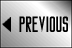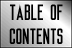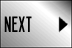STAR FLEET BATTLES ERRATA

## Star Fleet Battles Errata: (M2.111) through (M9.15)

(M2.111) Reference to (J1.5) should be to (J1.502).

(M2.131) Reference to (R10.1D4) should be to (R10.1D42).

(M2.21) Reference to (P8.0) should be to (P8.472). Reference to (P4.1) should be to (P4.14).

(M2.22) Reference (P5.35) should be (P5.23). Reference to (P9.317) should be to (P9.315). Reference to (P4.1) should be to (P4.21). (M2.34) See (C13.19) for additional information.

(M2.40) Either nimbleness or cloaking may protect a slow ship from detonating every mine. Movement of the mine itself will not cause the mine to trigger, only movement of a valid target.

(M2.41) While an anti-drone is, technically, a physical object, the passage of an anti-drone round through the detection range of a mine will not detonate the mine.

(M2.413) This example uses a detection radius of 1 hex.

(M2.414) Moving without expending movement points (e.g., pulled by a black hole) can trigger a mine (C2.451). Last line: also see (M2.43). (M2.416) Appearing by transporter (G19.41) will not, in itself, trigger a mine.

(M2.45) Cloaked minesweepers do not receive this benefit. Reference to (X0.0) should be to (XM2.45).

(M2.47) Example: First reference to (M2.44) should be to (M2.441). Second reference to (M2.44) should be to (M2.442).

(M2.6) Reference to (M3.226) should be to (M3.222).

(M2.61) The player must also record the settings (M2.14) and (M2.15). (M2.78) Add reference to (S3.27). Reference to (M9.21) should be to (M9.11).

(M2.81) See (G18.75) and (G19.49).

(M2.85) Reference to (M5.112) should be to (M5.1122). Reference to (M7.5) should be to (M7.51).

(M3.13) Reference to (G25.0) should be to (G25.13).

(M3.14) Stored T-bombs [and NSMs (M2.7)] are destroyed by the last Excess Damage hit; see (FD2.423).

(M3.22) EXCEPTION: (G13.616).

(M3.221) Reference to (G8.11) should be to (G8.112). Reference to (D21.32) should be to (D21.31).

(M3.224) Dummy T-bombs come ONLY with T-bombs purchased as part of Commander's Options. Dummy T-bombs cannot, under ANY circumstances, be replaced with real T-bombs.

(M4.4252) TYPO: "..to a maximum range of 35 hexes from the MINE." (M4.427) Reference to (M5.28) should be to (M8.42).

(M5.1121) Reference to (M2.45) should be to (M2.85).

(M5.1123) Reference to (G18.0) should be to (G18.65). Reference to (G19.4) should be to (G19.41).

(M5.20) A given mine can accept any number of orders in a single impulse.

(M5.34) Two chain mines, two deadman mines, or one of each would violate this rule.

(M6.1) The data set in this rule must conform to (M5.33); the phrase "size or distance" is irrelevant. Change text saying "hidden placement" to read "hidden deployment" with a cross reference to (D20.0).

(M6.33) There is no limit to the number of mine "belts" that might be deployed around a base, but it would not be a good investment of BPV. (M6.331) As per (M6.2), captor and sensor mines can be up to six hexes from the belt.

(M7.11) Add note that probes and probe drones cannot detect minefields, but can identify individual mines under (M7.52).

(M7.12) The distance given is to the ship that detected the minefield.

(M7.323) Add "at up to six hexes (J9.12)" to the end.

(M7.52) Note that a probe drone that reaches the hex of a mine can identify that mine as a probe under (G5.25).

(M8.11) A unit that is unable to move can sweep mines adjacent to itself under the provisions of this rule.

(M8.21) The unit launching the seeking weapon at the mine can be moving at any speed from zero to thirty-one hexes per turn.

(M8.24) The (D6.361) penalty does not apply to type-VI drones.

(M8.312) Minelaying freighters do not have MSS shuttles, but use MLS shuttles instead.

(M8.313) It is theoretically possible for an MSS to sweep 32 mines in a single turn provided it began that turn in a hex with 32 mines, or was able to move to hexes with more mines on each of its movements.

(M8.32) The "5" result applies even for mines that would not normally explode, since this is the anti-tamper "salvage" charge.

(M8.6) Reference to (M8.2) should be (M8.14).

(M9.15) Small explosive mines removed from the mine racks (M9.19) can also be used as T-bombs on the following turn.